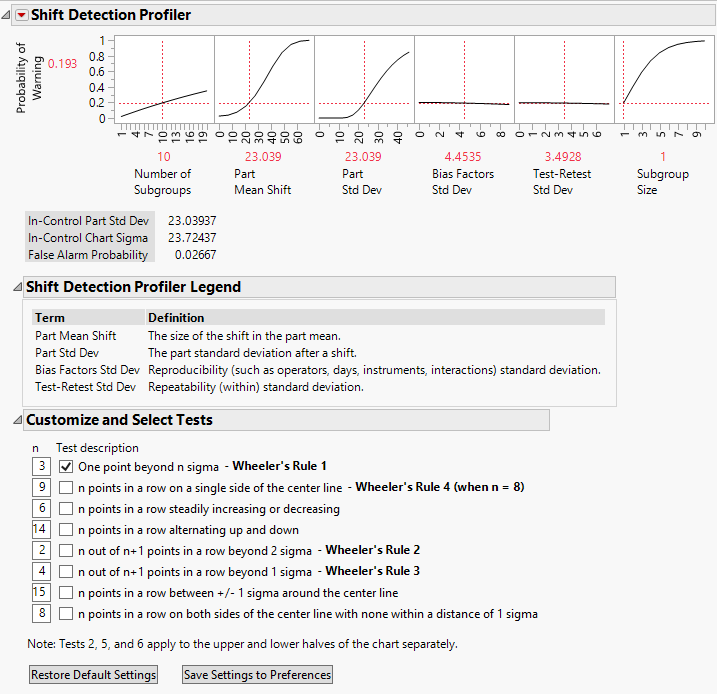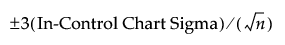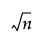Publication date: 11/10/2021

## Shift Detection Profiler

Use the Shift Detection Profiler to assess the sensitivity of the control chart that you use to monitor your process. The Shift Detection Profiler estimates the probability of detecting shifts in the product mean or product standard deviation. The control chart limits include sources of measurement error variation. Based on these limits, the Shift Detection Profiler estimates the Probability of Warning. This is the probability that a control chart monitoring the process mean signals a warning over the next k subgroups.

You can set the subgroup size that you want to use for your control chart. Note the following:

If the Subgroup Size equals one, the control chart is an Individual Measurement chart.

If the Subgroup Size exceeds one, the control chart is an XBar-chart.

You can explore the effect of Subgroup Size on the control chart’s sensitivity. You can also explore the benefits of reducing bias and test-retest error.

Figure 4.7 shows the Shift Detection Profiler report for the Gasket.jmp sample data table, found in the Variability Data folder.

Figure 4.7 Shift Detection Profiler for Gasket.jmp#### Probability of Warning

The Probability of Warning is the probability of detecting a change in the process. A change is defined by the Part Mean Shift and the Part Std Dev settings in the Shift Detection Profiler. The probability calculation assumes that the tests selected in the Customize and Select Tests outline are applied to the Number of Subgroups specified in the Profiler.

The control limits for the Individual Measurement chart (Subgroup Size = 1) and the XBar-chart (Subgroup Size > 1) are based on the In-Control Chart Sigma. The In-Control Sigma takes into account the bias factor (reproducibility) variation and the test-retest (repeatability) variation. These are initially set to the values obtained from your MSA study. The In-Control Chart Sigma also incorporates the In-Control Part Std Dev. Both of these values appear beneath the profiler, along with the False Alarm Probability, which is based on the In-Control Chart Sigma.

In-Control Part Std Dev

The standard deviation for the true part values, exclusive of measurement errors, for the stable process. The default value for In-Control Part Std Dev is the standard deviation of the part component estimated by the MSA analysis and found in the Variance Components report.

Often, parts for an MSA study are chosen to have specific properties and do not necessarily reflect the part-to-part variation seen in production. For this reason, you can specify the in-control part standard deviation by selecting Change In-Control Part Std Dev from the Shift Detection Profiler red triangle menu.

In-Control Chart Sigma

The value of sigma used to compute control limits. This value is computed using the In-Control Part Std Dev, the Bias Factors Std Dev, and Test-Retest Std Dev specified in the Shift Detection Profiler, and the Subgroup Size. The reproducibility factors are assumed to be constant within a subgroup.

For a subgroup of size n, control limits are set at the following values:It follows that the In-Control Chart Sigma is the square root of the sum of the squares of the following terms:

In-Control Part Std Dev

Bias Factors Std Dev, as specified in the Shift Detection Profiler, multiplied byTest-Retest Std Dev, as specified in the Shift Detection Profiler

The Bias Factors Std Dev is multiplied byto account for the assumption that the reproducibility factors are constant within a subgroup.

JMP updates the In-Control Chart Sigma when you change the In-Control Part Std Dev, the Bias Factors Std Dev, the Test-Retest Std Dev, or the Subgroup Size.

False Alarm Probability

The probability that the control chart tests signal a warning when no change in the part mean or standard deviation has occurred. JMP updates the False Alarm Probability when you change the Number of Subgroups or the tests in Customize and Select Tests.

### Shift Detection Profiler Settings

Number of Subgroups

The number of subgroups over which the probability of a warning is computed. If the number of subgroups is set to k, the profiler gives the probability that the control chart signals at least one warning based on these k subgroups. The Number of Subgroups is set to 10 by default. Drag the vertical line in the plot to change the Number of Subgroups.

Part Mean Shift

The shift in the part mean. By default, the profiler is set to detect a 1 sigma shift. The initial value is the standard deviation of the part component estimated by the MSA analysis and found in the Variance Components report. Drag the vertical line in the plot or click the value beneath the plot to change the Part Mean Shift.

Part Std Dev

The standard deviation for the true part values, exclusive of measurement errors. The initial value for Part Std Dev is the standard deviation of the part component estimated by the MSA analysis and is found in the Variance Components report. Drag the vertical line in the plot or click the value beneath the plot to change the Part Std Dev.

Bias Factors Std Dev

The standard deviation of factors related to reproducibility. Bias factors include operator and instrument. The bias factor variation does not include part and repeatability (within) variation. The initial value is derived using the reproducibility and interaction variance components estimated by the MSA analysis and is found in the Variance Components report. Drag the vertical line in the plot or click the value beneath the plot to change the Bias Factors Std Dev.

Test-Retest Std Dev

The standard deviation of the test-retest, or repeatability, variation in the model. The initial value is the standard deviation of the Within component estimated by the MSA analysis and is found in the Variance Components report. Drag the vertical line in the plot or click the value beneath the plot to change the Test-Retest Std Dev.

Subgroup Size

The sample size used for each subgroup. This is set to 1 by default. You can increase the sample size to investigate improvement in control chart performance. Increasing the sample size from 1 demonstrates what happens when you move from an Individual Measurement chart to an XBar-chart. Drag the vertical line in the plot to change the Subgroup Size.

### Shift Detection Profiler Options

The red triangle menu for the Shift Detection Profiler provides several options. Only one option is described here.

Change In-Control Part Std Dev

Specify a value for the part standard deviation for the stable process. The in-control part standard deviation should reflect the variation of the true part values, exclusive of measurement errors. Enter a new value and click OK.

The In-Control Part Std Dev is originally set to the standard deviation of the part component estimated by the MSA analysis, found in the Variance Components report.

This option is useful if the parts chosen for the EMP study were not a random sample from the process.

Reset Factor Grid

Displays a window for each factor allowing you to enter a specific value for the factor’s current setting, to lock that setting, and to control aspects of the grid. See Set or Lock Factor Values in Profilers.

Factor Settings

Submenu that consists of the following options:

Remember Settings

Adds an outline node to the report that accumulates the values of the current settings each time the Remember Settings command is invoked. Each remembered setting is preceded by a radio button that is used to reset to those settings. There are options to remove selected settings or all settings in the Remember Settings red triangle menu.

Copy Settings Script

Copies the current Profiler’s settings to the clipboard.

Paste Settings Script

Pastes the Profiler settings from the clipboard to a Profiler in another report.

Set Script

Sets a script that is called each time a factor changes. The set script receives a list of arguments of the form:

`	{factor1 = n1, factor2 = n2, ...}`

For example, to write this list to the log, first define a function:

`	ProfileCallbackLog = Function({arg},show(arg));`

Similar functions convert the factor values to global values:

`	ProfileCallbackAssign = Function({arg},evalList(arg));`

Or access the values one at a time:

ProfileCallbackAccess = Function({arg},f1=arg["factor1"];f2=arg["factor2"]);

### Shift Detection Profiler Legend

This panel gives a brief description of four of the Shift Detection Profiler settings. See Shift Detection Profiler Settings.

Tip: To prevent the legend from appearing, deselect Show Shift Detection Profiler Legend in the EMP Measurement Systems Analysis platform preferences.

### Customize and Select Tests

In the Customize and Select Tests panel, select and customize the tests that you want to apply to the k subgroups in your control chart. The eight tests are based on Nelson (1984). For more information about the tests, see Tests.

The Shift Detection Profiler calculations take these tests into account. The Probability of Warning and False Alarm Probability values increase as you add more tests. Because the calculations are based on a quasi-random simulation, there might be a slight delay as the profiler is updated.

The Customize and Select Tests panel has the following options:

Restore Default Settings

If no settings have been saved to preferences, this option resets the selected tests to the first test only. The values of n are also reset to the values described in Tests. If settings have been saved to preferences, this option resets the selected tests and the values of n to those specified in the preferences.

Note: You can access preferences for control chart tests by selecting File > Preferences > Platforms > Control Chart Builder. Custom Tests 1 through 8 correspond to the eight tests shown in Customize and Select Tests.

Save Settings to Preferences

Saves the selected tests and the values of n for use in future analyses. These preferences are added to the Control Chart Builder platform preferences.